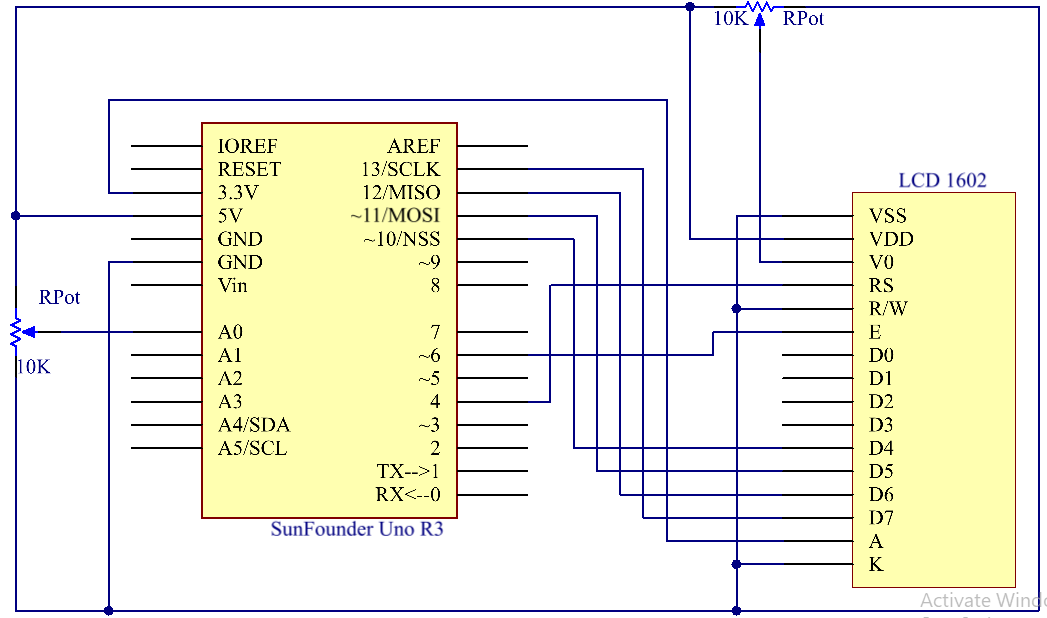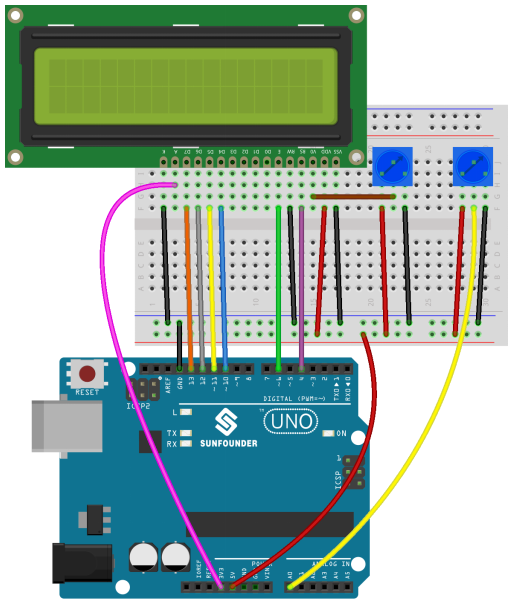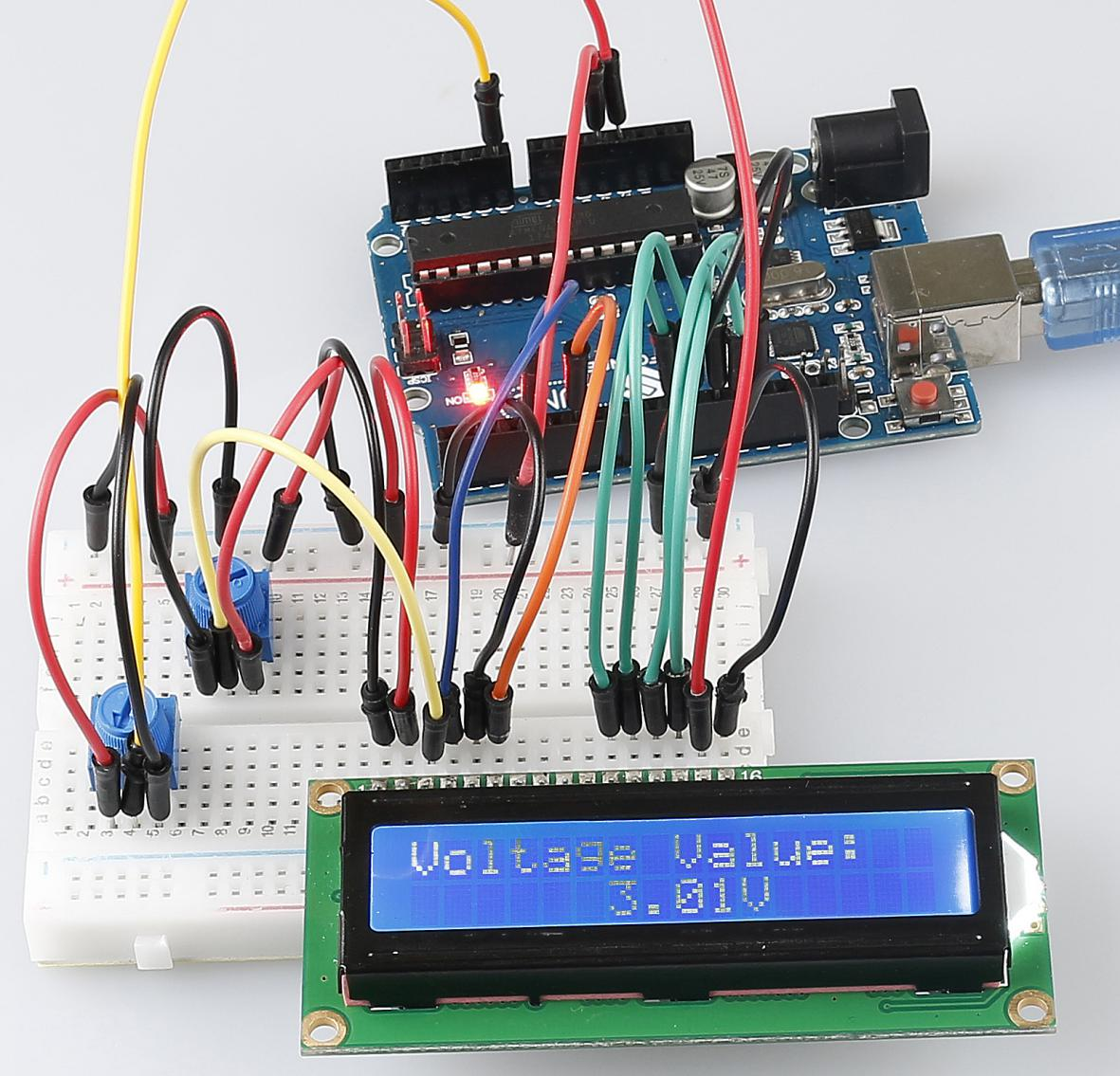# Lesson 15 Voltmeter¶

## Introduction¶

In this lesson, we will use two potentiometers and an LCD1602 to make a DIY voltmeter.

## Experimental Principle¶

Here one potentiometer is used to adjust the contrast of the LCD1602 and the other to divide voltage. When you adjust the potentiometer connected to pin A0 of the SunFounder Uno board, the resistance of the potentiometer will change and the voltage at pin A0 will change accordingly. This voltage change is converted into digital values by A/D converter on the SunFounder Uno board. We can see this change on the serial monitor. Then convert the digital values into voltage with the following formula: the voltage equals the digital value divides by 1024 and then multiplies by 5.0. Finally, display the voltage on the LCD1602.

The schematic diagram:## Experimental Procedures¶

Step 1: Build the circuit

Step 2: Open the code file

Step 3: Select the correct Board and Port

Step 4: Upload the sketch to the SunFounder Uno boardNow, adjust the potentiometer connected to pin A0, and you will see the voltage displayed on the LCD1602 varies accordingly.## Code¶

### Code Analysis15-1Define the pins of LCD1602 andpotentiometer¶

```#include <LiquidCrystal.h>

/****************************************************/

float analogIn = 0;                      // store the analog value of A0

LiquidCrystal lcd(4, 6, 10, 11, 12, 13); // lcd(RS,E,D4,D5,D6.D7)

float vol = 0;                           // store the voltage
```

Call the LiquidCrystal library and define the pins of LCD1602 connect to 4,6 and 10 to 13 of Uno board.

Assign the value of A0 to analogIn.

### Code Analysis15-2Initialize the LCD1602 and serial monitor¶

```void setup()

{

Serial.begin(9600);          // Initialize the serial monitor

lcd.begin(16, 2);            // set the position of the characters on the LCD as Line 2, Column 16

lcd.print("Voltage Value:"); // print "Voltage Value:"

}
```

Initialize the baud rate of serial monitor to 9600bps and set the position of the characters on the LCD as Line 2, Column 16. Print “Voltage Value: ” on the LCD1602.

### Code Analysis15-3Read the analog of A0 and convert to voltage¶

```void loop()

{

vol = analogIn/1024*5.0;     // Convert the data to the corresponding voltage value in a math way

Serial.print(vol);           // Print the number of val on the serial monitor

Serial.println("V");       // print the unit as V, short for voltage on the serial monitor

lcd.setCursor(6,1);      // Place the cursor at Line 1, Column 6. From here the characters are to be displayed

lcd.print(vol);             // Print the number of val on the LCD

lcd.print("V");            // Then print the unit as V, short for voltage on the LCD

delay(200);                // Wait for 200ms

}
```

The analog value of A0 is: Analog value=5/VA0 * 1024, so VA0= Analog value/1024 * 5, if you connect the potentiometer to 3.3v, then modify 5V to 3.3V.

Print the voltage to serial monitor or the LCD1602.